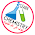## Breaking News

#### Atomic Mass

** The mass of an atom depends on the number of electrons, protons, and neutrons it contains.

** Knowledge of an atom’s mass is important in laboratory work. But atoms are extremely small particles—even the smallest speck of dust that our unaided eyes can detect contains as many as 1 × 1016 atoms! Clearly we cannot weigh a single atom, but it is possible to determine the mass of one atom relative to another experimentally.

** The first step is to assign a value to the mass of one atom of a given element so that it can be used as a standard.

** By international agreement, atomic mass (sometimes called atomic weight) is the mass of the atom in atomic mass units (amu).

** One atomic mass unit (amu) is defined as a mass exactly equal to one-twelfth the mass of one carbon-12 atom. Carbon-12 is the carbon isotope that has six protons and six neutrons. Setting the atomic mass of carbon-12 at 12 amu provides the standard for measuring the atomic mass of the other elements.

** For example, experiments have shown that, on average, a hydrogen atom is only 8.400 percent as massive as the carbon-12 atom. Thus, if the mass of one carbon-12 atom is exactly 12 amu, the atomic mass of hydrogen must be 0.084 × 12.00 amu or 1.008 amu.

** Similar calculations show that the atomic mass of oxygen is 16.00 amu and that of iron is 55.85 amu. Thus, although we do not know just how much an average iron atom’s mass is, we know that it is approximately 56 times as massive as a hydrogen atom.

Note: One atomic mass unit (1 amu) is also called one dalton.

#### Average Atomic Mass

** When you look up the atomic mass of carbon in a table such as the one on the inside front cover of this book, you will find that its value is not 12.00 amu but 12.01 amu.

** The reason for the difference is that most naturally occurring elements (including carbon) have more than one isotope.

** This means that when we measure the atomic mass of an element, we must generally settle for the average mass of the naturally occurring mixture of isotopes.

** For example, the natural abundances of carbon-12 and carbon-13 are 98.90 percent and 1.10 percent, respectively. The atomic mass of carbon-13 has been determined to be 13.00335 amu. Thus, the average atomic mass of carbon can be calculated as follows:

** Note that in calculations involving percentages, we need to convert percentages to fractions. For example, 98.90 percent becomes 98.90/100, or 0.9890. Because there are many more carbon-12 atoms than carbon-13 atoms in naturally occurring carbon, the average atomic mass is much closer to 12 amu than to 13 amu.

** It is important to understand that when we say that the atomic mass of carbon is 12.01 amu, we are referring to the average value. If carbon atoms could be examined individually, we would find either an atom of atomic mass 12.00000 amu or one of 13.00335 amu, but never one of 12.01 amu.

#### Examples

Example: Copper, a metal known since ancient times, is used in electrical cables and pennies, among other things. The atomic masses of its two stable isotopes, 63Cu29 (69.09 percent) and 65Cu29 (30.91 percent), are 62.93 amu and 64.9278 amu, respectively. Calculate the average atomic mass of copper. The relative abundances are given in parentheses.

Strategy
Each isotope contributes to the average atomic mass based on its relative abundance. Multiplying the mass of an isotope by its fractional abundance (not percent) will give the contribution to the average atomic mass of that particular isotope.

Solution
First the percents are converted to fractions: 69.09 percent to 69.09y100 or 0.6909 and 30.91 percent to 30.91y100 or 0.3091. We find the contribution to the average atomic mass for each isotope, then add the contributions together to obtain the average atomic mass.

Check
The average atomic mass should be between the two isotopic masses; therefore, the answer is reasonable. Note that because there are more 63Cu29 than 65Cu29isotopes, the average atomic mass is closer to 62.93 amu than to 64.9278 amu.

Practice Exercise: The atomic masses of the two stable isotopes of boron, 10B5 (19.78 percent) and 11B5 (80.22 percent), are 10.0129 amu and 11.0093 amu, respectively. Calculate the average atomic mass of boron?

#### Notes

** The atomic masses of many elements have been accurately determined to five or six significant figures. However, for our purposes we will normally use atomic masses accurate only to four significant figures

** For simplicity, we will omit the word “average” when we discuss the atomic masses of the elements.

** Table shows the atomic masses of elements:

Reference: General Chemistry: The Essential Concepts / Raymond Chang , Jason Overby. (sixth edition).

1.1.